# Maharashtra Board Class 11 Chemistry Solutions Chapter 5 Chemical Bonding

Balbharti Maharashtra State Board 11th Chemistry Textbook Solutions Chapter 5 Chemical Bonding Textbook Exercise Questions and Answers.

## Maharashtra State Board 11th Chemistry Solutions Chapter 5 Chemical Bonding

1. Select and write the most appropriate alternatives from the given choices.

Question A.
Which molecule is linear?
a. SO3
b. CO2
c. H2S
d. Cl2O
b. CO2

Question B.
When the following bond types are listed in decreasing order of strength (strongest first). Which is the correct order?
a. covalent > hydrogen > van der waals
b. covalent > vander waal’s > hydrogen
c. hydrogen > covalent > vander waal’s
d. vander waal’s > hydrogen > covalent.
a. covalent > hydrogen > van der waals

Question C.
Valence Shell Electron Pair repulsion (VSEPR) theory is used to predict which of the following :
a. energy levels in an atom
b. the shapes of molecules and ions.
c. the electron negetivities of elements.
d. the type of bonding in compounds.
b. the shapes of molecules and ions.

Question D.
Which of the following is true for CO2?

 C=O bond CO2 molecule A polar non-polar B non-polar polar C polar polar D non-polar non-polar

 C=O bond CO2 molecule A polar non-polarQuestion E.
Which O2 molecule is pargmagnetic. It is explained on the basis of :
a. Hybridisation
b. VBT
c. MOT
d. VSEPR
c. MOT

Question F.
The angle between two covalent bonds is minimum in:
a CH4
b. C2H2
c. NH3
d. H2O
d. H2O

2. Draw

Question A.
Lewis dot diagrams for the folowing
a. Hydrogen (H2)
b. Water (H2O)
c. Carbon dioxide (CO2)
d. Methane (CH4)
e. Lithium Fluoride (LiF)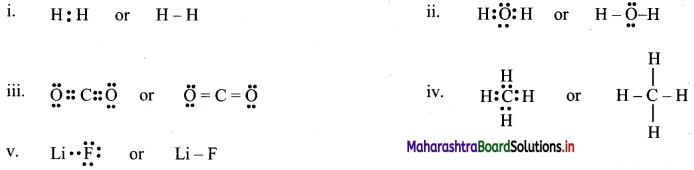[Note: H atom in H2 and Li atom in LiF attain the configuration of helium (a duplet of electrons).]Question B.
Diagram for bonding in ethene with sp2 Hybridisation.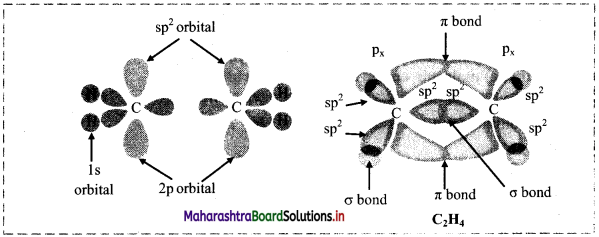Question C.
Lewis electron dot structures of
a. HF
b. C2H6
c. C2H4
d. CF3Cl
e. SO2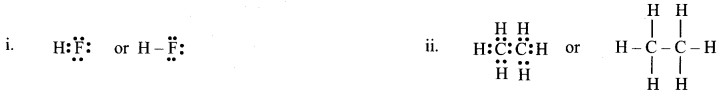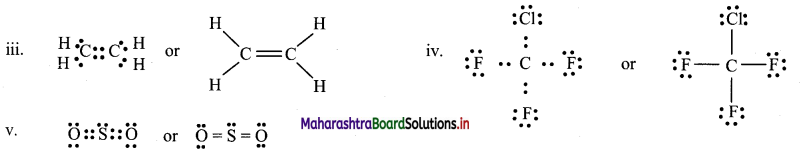Question D.
Draw orbital diagrams of
a. Fluorine molecule
b. Hydrogen fluoride molecule
a.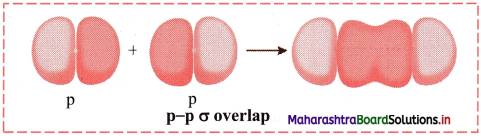b.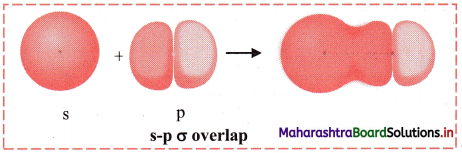Question A.
Distinguish between sigma and pi bond.

 σ (sigma) bond π (pi) bond 1. It is formed when atomic orbitals overlap along internuclear axis. 1. It is formed when atomic orbitals overlap side-ways (laterally). 2. Electron density is high along the axis of the molecule (i.e., internuclear axis). 2. Electron density is zero along the axis of the molecule (i.e., internuclear axis). 3. In the formation of sigma bond, the extent of overlap is greater, hence, more energy is released. 3. In the formation of pi bond, the extent of overlap is less, hence, less energy is released. 4. It is a strong bond. 4. It is a weak bond. 5. Formation of sigma bonds involves s-s, s-p, p-p overlap and overlap between hybrid orbitals. 5. Formation of pi bonds involves p-p or d-d overlap. The overlap between hybrid orbitals is not involved.Question B.
Display electron distribution around the oxygen atom in water molecule and state shape of the molecule, also write H-O-H bond angle.
Electron distribution around oxygen atom in water molecule:
Shape of water molecule: Angular or V shaped H-O-H bond angle = 104°35′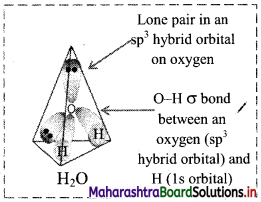Question C.
State octet rule. Explain its inadequecies with respect to
a. Incomplete octet
b. Expanded octet
Statement: During the formation of chemical bond, atom loses, gains or shares electrons so that its outermost orbit (valence shell) contains eight electrons. Therefore, the atom attains the nearest inert gas electronic configuration.

a. Molecules with incomplete octet: e.g. BF3, BeCl2, LiCl
In these covalent molecules, the atoms B, Be and Li have less than eight electrons in their valence shell but these molecules are stable.
Li in LiCl has only two electrons, Be in BeCl2 has four electrons while B in BF3 has six electrons in the valence shell.

b. Molecules with expanded octet: Some molecules like SF6, PCl5, H2SO4 have more than eight electrons around the central atom.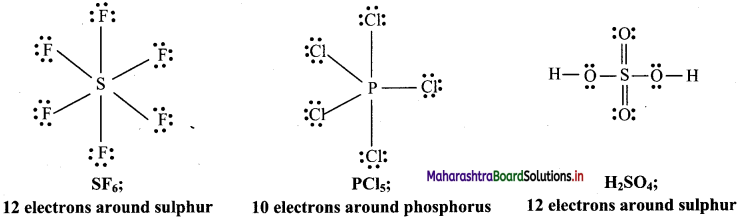Question D.
Explain in brief with one example:
a. Ionic bond
b. covalent bond
c. co-ordinate bond
a. Formation of calcium chloride (CaCl2):
i. The electronic configurations of calcium and chlorine are:
Na (Z = 11): 1s2 2s2 2p6 3s2 3p6 4s2 or (2, 8, 8, 2)
Cl (Z = 17): 1s2 2s2 2p6 3s2 3p5 or (2, 8, 7)
ii. Calcium has two electrons in its valence shell. It has tendency to lose two electrons to acquire the electronic configuration of the nearest inert gas, argon (2, 8, 8).
iii. Chlorine has seven electrons in its valence shell. It has tendency to gain one electron and thereby acquire the electronic configuration of the nearest inert gas, argon (2, 8, 8).
iv. During the combination of calcium and chlorine atoms, the calcium atom transfers its valence electrons to two chlorine atoms.
v. Calcium atom changes into Ca2+ ion while the two chlorine atoms change into two Cl ions. These ions are held together by strong electrostatic force of attraction.
vi. The formation of ionic bond(s) between Ca and Cl can be shown as follows: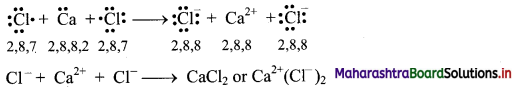b. Formation of Cl2 molecule:
i. The electronic configuration of Cl atom is [Ne] 3s2 3p5.
ii. It needs one more electron to complete its valence shell.
iii. When two chlorine atoms approach each other at a certain internuclear distance, they share their valence electrons. In the process, both the atoms attain the valence shell of octet of nearest noble gas, argon.
iv. The shared pair of electrons belongs equally to both the chlorine atoms. The two atoms are said to be linked by a single covalent bond and a Cl2 molecule is formed.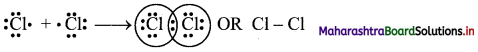c. co-ordinate bond:
i. A coordinate bond is a type of covalent bond where both of the electrons that form the bond originate from the same atom
ii. An atom with a lone pair of electrons (non-bonding pair of electrons) is capable of forming a coordinate bond.
iii. For example, reaction of ammonia with boron trifluoride: Before the reaction, nitrogen (N) in ammonia has eight valence electrons, including a lone pair of electrons. Boron (B) in boron trifluoride has only six valence electrons, so it is two electrons short of an octet. The two unpaired electrons form a bond between nitrogen and boron, resulting in complete octets for both atoms. A coordinate bond is represented by an arrow. The direction of the arrow indicates that the electrons are moving from nitrogen to boron. Thus, ammonia forms a coordinate bond with boron trifluoride.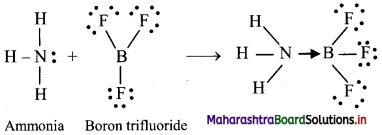iv. Once formed, a coordinate covalent bond is the same as any other covalent bond.

Question E.
Give reasons for need of Hybridisation.
The concept of hybridization was introduced because the valence bond theory failed to explain the following points:
i. Valencies of certain elements:
The maximum number of covalent bonds which an atom can form equals the number of unpaired electrons present in its valence shell. However, valence bond theory failed to explain how beryllium, boron and carbon forms two, three and four covalent bonds respectively.
a. Beryllium: The electronic configuration of beryllium is 1s2 2s2. The expected valency is zero (as there is no unpaired electron) but the observed valency is 2 as in BeCl2.
b. Boron: The electronic configuration of boron is 1s2 2s2 $$2 \mathrm{p}_{\mathrm{x}}^{1}$$. The valency is expected to be 1 but it is 3 as in BF3.
c. Carbon: The electronic configuration of carbon is 1s2 2s2 $$2 \mathrm{p}_{\mathrm{x}}^{1}$$ $$2 \mathrm{p}_{\mathrm{y}}^{1}$$ . The valency is expected to be 2, but observed valency is 4 as in CH4.

ii. The shapes and geometry of certain molecules:
The valence bond theory cannot explain shapes, geometries and bond angles in certain molecules,
e.g. a. Tetrahedral shape of methane molecule.
b. Bond angles in molecules like NH3 (107°18′) and H2O (104°35′).
However, the valency of the above elements and the observe structural properties of the above molecules can be explained by the concept of hybridization. These are the reasons for need of the concept of hybridization.Question F.
Explain geometry of methane molecule on the basis of Hybridisation.
Formation of methane (CH4) molecule on the basis of sp3 hybridization:
i. Methane molecule (CH4) has one carbon atom and four hydrogen atoms.
ii. The ground state electronic configuration of C (Z = 6) is 1s2 $$2 \mathrm{p}_{\mathrm{x}}^{1}$$ $$2 \mathrm{p}_{\mathrm{y}}^{1}$$ $$2 \mathrm{p}_{\mathrm{z}}^{1}$$;
Electronic configuration of carbon: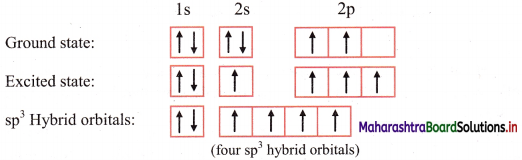iii. In order to form four equivalent bonds with hydrogen, the 2s and 2p orbitals of C-atom undergo sp3 hybridization.
iv. One electron from the 2s orbital of carbon atom is excited to the 2pz orbital. Then the four orbitals 2s, px, py and pz mix and recast to form four new sp3 hybrid orbitals having same shape and equal energy. They are maximum apart and have tetrahedral geometry with H-C-H bond angle of 109°28′. Each hybrid orbital contains one unpaired electron.
v. Each of these sp3 hybrid orbitals with one electron overlap axially with the 1s orbital of hydrogen atom to form one C-H sigma bond. Thus, in CH4 molecule, there are four C-H bonds formed by the sp3-s overlap.
Diagram: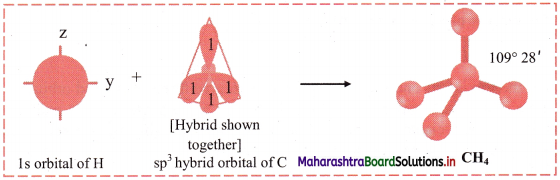Question G.
In Ammonia molecule the bond angle is 107°18 and in water molecule it is 104°35′, although in both the central atoms are sp3 hybridized Explain.
i. The ammonia molecule has sp3 hybridization. The expected bond angle is 109°28′. But the actual bond angle is 107°28′. It is due to the following reasons.

• One lone pair and three bond pairs are present in ammonia molecule.
• The strength of lone pair-bond pair repulsion is much higher than that of bond pair-bond pair repulsion.
• Due to these repulsions, there is a small decrease in bond angle (~2°) from 109°28′ to 107°18′.

ii. The water molecule has sp3 hybridization. The expected bond angle is 109°28′. But the actual bond angle is 104°35′. It is due to the following reasons.

• Two lone pairs and two bond pairs are present in water molecule.
• The decreasing order of the repulsion is Lone pair-Lone pair > Lone pair-Bond pair > Bond pair-Bond pair.
• Due to these repulsions, there is a small decrease in bond angle (~5°) from 109°28′ to 104°35′.

Question H.
Give reasons for:
a. Sigma (σ) bond is stronger than Pi (π) bond.
b. HF is a polar molecule
c. Carbon is a tetravalent in nature.
a. i. The strength of the bond depends on the extent of overlap of the orbitals. Greater the overlap, stronger is the bond.
ii. A sigma bond is formed by the coaxial overlap of the atomic orbitals which are oriented along the internuclear axis, hence the extent of overlap is maximum.
iii. A pi bond is formed by the lateral overlap of the atomic orbitals which are oriented perpendicular to the internuclear axis, hence the extent of orbital overlapping in side wise manner is less.
Hence, sigma bond is stronger than pi bond.

b. i. When a covalent bond is formed between two atoms of different elements that have different electronegativities, the shared electron pair does not remain at the centre. The electron pair is pulled towards the more electronegative atom resulting in the separation of charges.
ii. In H-F, fluorine is more electronegative than hydrogen. Therefore, the shared electron pair is pulled towards fluorine and fluorine acquires partial -ve charge and simultaneously hydrogen acquires partial +ve charge. This gives rise to dipole and H-F bond becomes polar. Hence, H-F is a polar molecule.

c. The electronic configuration of carbon is:
1s2 2s2 2px1 2py1
One electron from ‘2s’ orbital is promoted to the empty ‘2p’ orbital.
Thus, in excited state, carbon has four half-filled orbitals.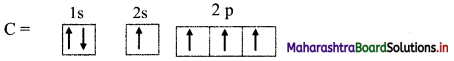Hence, carbon can form 4 bonds and is tetravalent in nature.

Question I.
Which type of hybridization is present in ammonia molecule? Write the geometry and bond angle present in ammonia.
The type of hybridization present in ammonia (NH3) molecule is sp3.
Geometry of ammonia molecule is pyramidal or distorted tetrahedral.
Bond angle in ammonia molecule is 107°18′.Question J.
Identify the type of orbital overlap present in
a. H2
b. F2
c. H-F molecule.
Explain diagramatically.
i. s-s σ overlap:
a. The overlap between two half-filled s orbitals of two different atoms containing unpaired electrons with opposite spins is called s-s overlap.
e.g. Formation of H2 molecule by s-s overlap:
Hydrogen atom (Z = 1) has electronic configuration: 1s1. The 1s1 orbitais of two hydrogen atoms overlap along the internuclear axis to form a σ bond between the atoms in H2 molecule.
b. Diagram: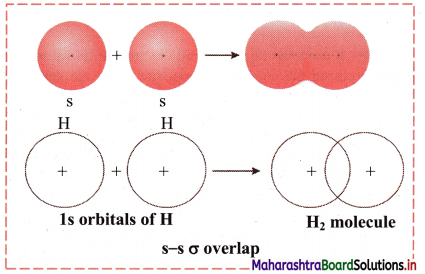ii. p-p σ overlap:
a. This type of overlap takes place when two p orbitals from different atoms overlap along the internuclear axis.
e.g. Formation of F2 molecule by p-p overlap:
Fluorine atom (Z = 9) has electronic configuration 1s2 2s2 $$2 \mathrm{p}_{\mathrm{x}}^{2}$$ $$2 \mathrm{p}_{\mathrm{y}}^{2}$$ $$2 \mathrm{p}_{\mathrm{z}}^{2}$$.
During the formation of F2 molecule, half-filled 2pz orbital of one F atom overlaps with similar half-filled 2pz orbital containing electron with opposite spin of another F atom axially and a p-p σ bond is formed.
b. Diagram: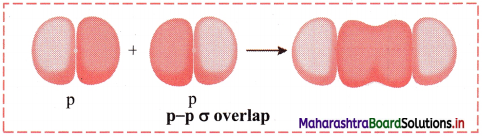iii. s-p σ overlap:
a. In this type of overlap one half filled s orbital of one atom and one half filled p orbital of another orbital overlap along the internuclear axis.
e.g. Formation of HF molecule by s-p overlap:
Hydrogen atom (Z = 1) has electronic configuration: 1s1 and fluorine atom (Z = 9) has electronic configuration 1s2 2s2 $$2 \mathrm{p}_{\mathrm{x}}^{2}$$ $$2 \mathrm{p}_{\mathrm{y}}^{2}$$ $$2 \mathrm{p}_{\mathrm{z}}^{2}$$. During the formation of HF molecule, half-filled Is orbital of hydrogen atom overlaps coaxially with half-filled 2pz orbital of fluorine atom with opposite electron spin and an s-p σ bond is formed.
b. Diagram: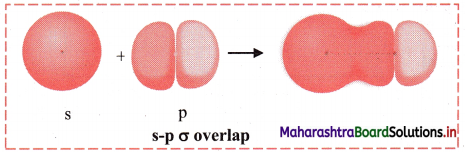Question K.
F-Be-F is a liner molecule but H-O-H is angular. Explain.
i. In the BeF2 molecule, the central beryllium atom undergoes sp hybridization giving rise to two sp hybridized orbitals placed diagonally opposite with an angle of 180°. Thus, F-Be-F is a linear molecule.

ii. In the H2O molecule, the central oxygen atom undergoes sp3 hybridization giving rise to four sp3 hybridized orbitals directed towards four comers of a tetrahedron. There are two lone pairs of electrons in two of the sp3 hybrid orbitals of oxygen. The lone pair-lone pair repulsion distorts the structure. Hence, H-O-H is angular or V-shaped.

Question L.
BF3 molecule is planar but NH3 pyramidal. Explain.
i. In the BF3 molecule, the central boron atom undergoes sp2 hybridization giving rise to three sp2 hybridized orbitals directed towards three comers of an equilateral triangle. Thus, the geometry is trigonal planar.

ii. In the NH3 molecule, the central nitrogen atom undergoes sp3 hybridization giving rise to four sp3 hybridized orbitals directed towards four comers of a tetrahedron. The expected geometry of NH3 molecule is regular tetrahedral with bond angle 109°28′. There is one lone pair of electrons in one of the sp3 hybrid orbitals of nitrogen. The lone pair-bond pair repulsion distorts the bond angle. Hence, the structure of NH3 is distorted and it has pyramidal geometry.

Question M.
In case of bond formation in Acetylene molecule :
a. How many covalend bonds are formed ?
b. State number of sigma and pi bonds formed.
c. Name the type of Hybridisation.
a. In acetylene molecule, there are five covalent bonds.
b. In acetylene molecule, there are three sigma bonds and two pi bonds.
c. In acetylene molecule, each carbon atom undergoes sp hybridization.

Question N.
Define :
a. Bond Enthalpy
b. Bond Length
a. Bond Enthalpy:
Bond enthalpy is defined as the amount of energy required to break one mole of a bond of one type, present between two atoms in a gaseous state.

b. Bond Length:
Bond length is defined as the equilibrium distance between the nuclei of two covalently bonded atoms in a molecule.Question O.
Predict the shape and bond angles in the following molecules:
a. CF4
b. NF3
c. HCN
d. H2S
a. CF4: There are four bond pairs on the central atom. Hence, shape of CF4 is tetrahedral and F-C-F bond angle is 109° 28′.
b. NF3: There are three bond pairs and one lone pair on the central atom. Hence, shape of NF3 is trigonal pyramidal and F-N-F bond angle is less than 109° 28′.
c. HCN: There are two bond pairs on the central atom. Hence, shape of HCN is linear and H-C-N bond angle is 180°.
d. H2S: There are two bond pairs and two lone pairs on the central atom. Hence, shape of H2S is bent or V-shaped and H-S-H bond angle is slightly less than 109° 28′.

4. Using data from the Table, answer the following :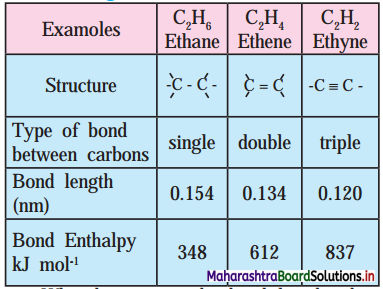a. What happens to the bond length when unsaturation increases?
b. Which is the most stable compound?
c. Indicate the relation between bond strength and Bond enthalpy.
d. Comment on overall relation between Bond length, Bond Enthalpy and Bond strength and stability.
a. When unsaturation increases, the bond length decreases.
b. The stable compound is ethyne (C2H2).
c. Bond strength ∝ Bond enthalpy
Larger the bond enthalpy, stronger is the bond.
d. As bond length decreases, bond enthalpy, bond strength and stability increase.5. Complete the flow chart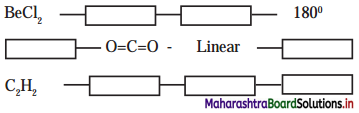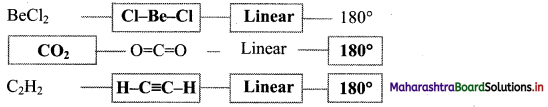6. Complete the following Table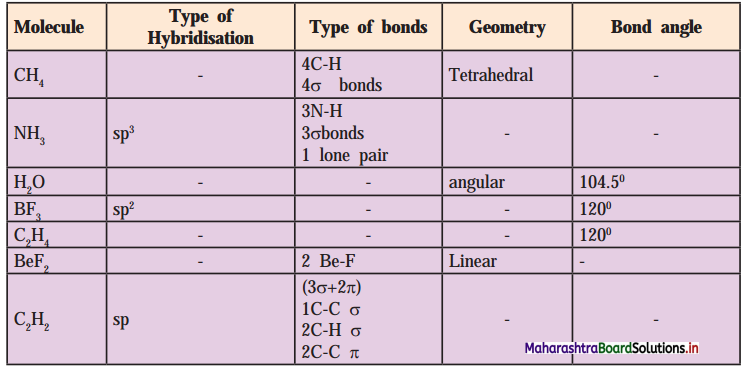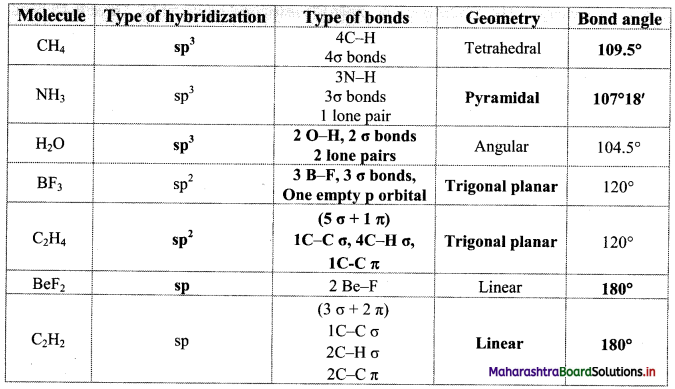Question A.
Indicate the factor on which stalility of ionic compound is measured?
The stability of an ionic compound is measured by the amount of energy released during lattice formation.

Question B.
Arrange the following compounds on the basis of lattice energies in decreasing (descending) order: BeF2, AlCl3, LiCl, CaCl2, NaCl.
AlCl3 > BeF2 > CaCl2 > LiCl > NaCl

Question C.
Give the total number of electrons around sulphur (S) in SF6 compound.
The total number of electrons around sulphur (S) in SF6 is 12.Question D.
Covalant bond is directional in nature. Justify.
Covalent bond is formed by the overlap of two half-filled atomic orbitals. The atomic orbitals are oriented in specific directions in space (except s-orbital which is spherical). Hence, covalent bond is directional in nature.

Question E.
What are the interacting forces present during formation of a molecule of a compound ?
a. Forces of attraction: The nucleus of one atom attracts the electrons of the other atom and vice-versa.
b. Forces of repulsion: The electron of one atom repels the electron of the other atom and vice-versa (as electrons are negatively charged). There is repulsion between the two nuclei (as the nuclei are positively charged).

Question F.
Give the type of overlap by which pi (π) bond is formed.
The type of overlap by which pi (π) bond is formed is p-p lateral overlap.

Question G .
Mention the steps involved in Hybridization.
The steps involved in hybridization are:

• formation of the excited state and
• mixing and recasting of orbitals.

Question H.
Write the formula to calculate bond order of molecule.
Bond order of a molecule = $$\frac{\mathrm{N}_{\mathrm{b}}-\mathrm{N}_{\mathrm{a}}}{2}$$
where, Nb is the number of electrons present in bonding MOs and Na is the number of electrons present in antibonding MOs.

Question I.
Why is O2 molecule paramagnetic?
The electronic configuration of O2 molecule is (σ1s)2 (σ*1s)2 (σ2s)2 (σ*2s)2 (σ2pz)2 (π2px)2 (π2py)2 (π*2px)1 (π*2py)1
Since the oxygen molecule contains two unpaired electrons, it is paramagnetic.Question J.
What do you mean by formal charge ? Explain its significance with the help of suitable example.
Formal charge is the charge assigned to an atom in a molecule, assuming that all electrons are shared equally between atoms, regardless of their relative electronegativities.

Structure (I):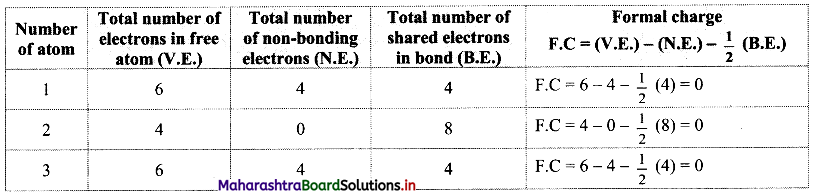Structure (II):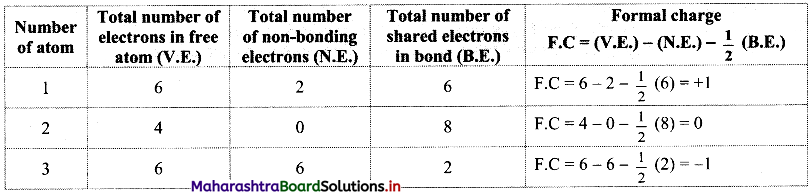Structure (III):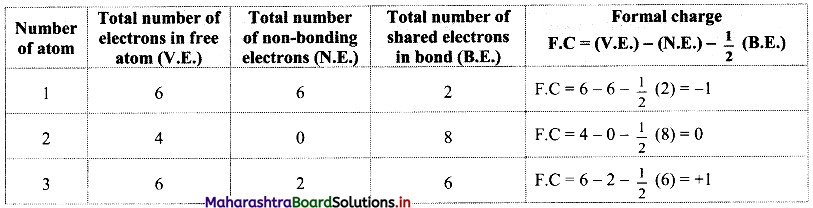While determining the best Lewis structure per molecule, the structure is chosen such that the formal charge is as close to zero as possible. The structure having the lowest formal charge has the lowest energy.

In structure (I), the formal charge on each atom is 0 while in structures (II) and (III) formal charge on carbon is 0 while oxygens have formal charge -1 or +1. Hence, the possible structure with the lowest energy will be structure (I). Thus, formal charges help in the selection of the lowest energy structure from a number of possible Lewis structures for a given species.

11th Chemistry Digest Chapter 5 Chemical Bonding Intext Questions and Answers

(Textbook Page No. 55)

Question 1.
Why are atoms held together in chemical compounds?
Atoms are held together in chemical compounds due to chemical bonds.

Question 2.
How are chemical bonds formed between two atoms?
There are two ways of formation of chemical bonds:

1. by loss and gain of electrons
2. by sharing a pair of electrons between the two atoms.

In either process of formation of chemical bond, each atom attains a stable noble gas electronic configuration.

Question 3.
Which electrons are involved in the formation of chemical bonds?
The electrons present in the outermost shell of an atom are involved in the formation of a chemical bond.Internet my friend (Textbook Page No. 55)

Question 1.
Search more atoms, which complete their octet during chemical combinations.
In compounds like KCl, MgCl2, CaO, NaF, etc, the constituent atoms complete their octet by lose or gain of electrons.
e.g. K → K+ + e
Cl + e → Cl
K+ + Cl → KCl
[Note: Students are expected to search more atoms on their own.]

Use your brainpower. (Textbook Page No. 60)

Question 1.
Which atom in $$\mathrm{NH}_{4}^{+}$$ will have formal charge +1?
In $$\mathrm{NH}_{4}^{+}$$, nitrogen atom (N) will have formal charge of+1.

Use your brainpower. (Textbook Page No. 61)

Question 1.
How many electrons will be around I in the compound IF7?
Lewis structure of IF7 is: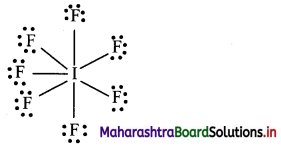In IF7, iodine (I) atom will be surrounded by 14 electrons.

Question 2.
Why is H2 stable even though it never satisfies the octet rule?
The valence shell configuration of hydrogen atom is 1s1. Two hydrogen atoms approach each other and share their valence electrons. By having two electrons in its valence shell, H atom attains the nearest noble gas configuration of He. H2 molecule attains stability due to duplet formation. Hence, H2 is stable even though it never satisfies the octet rule.(Textbook Page No. 64)

Question 1.
Lowering of energy takes during bond formation. How does this happen?
i. When two combining atoms approach each other to form a covalent bond, the following interacting forces come into play.

• Forces of attraction: The nucleus of one atom attracts the electrons of the other atom and vice-versa.
• Forces of repulsion: The electron of one atom repels the electron of the other atom and vice-versa (as electrons are negatively charged). There is repulsion between the two nuclei (as the nuclei are positively charged).

ii. The balance between attractive and repulsive forces decide whether the bond will be formed or not.
iii. When the magnitude of attractive forces is more than the magnitude of repulsive forces, the energy of the system decreases and a covalent bond is formed.
iv. When the magnitude of repulsive forces becomes more than that of attraction, the total energy of the system increases, and a covalent bond is not formed.
Hence, lowering of energy takes during bond formation.

Can you tell? (TextBook Page No. 76)

Question 1.
Which molecules are polar?
H-I, H-O-H, H-Br, Br2, N2, I2, NH3